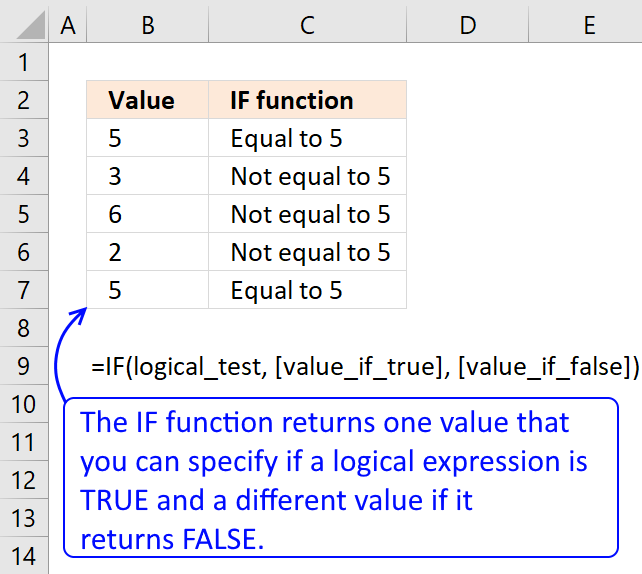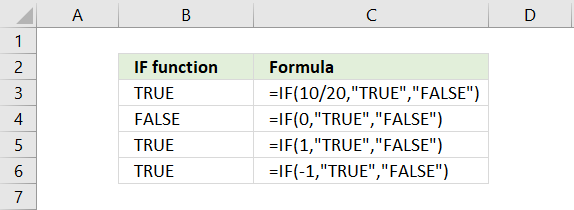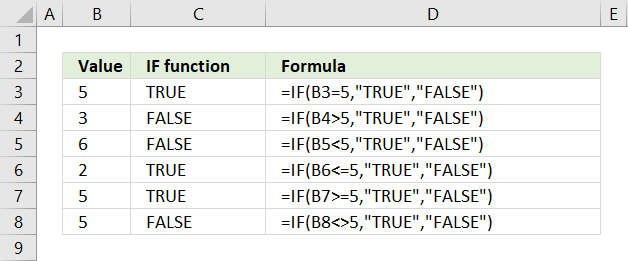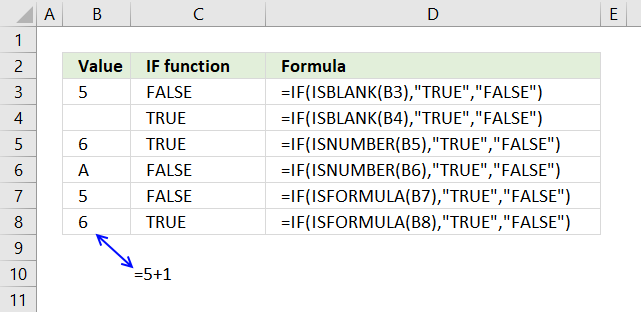Author: Oscar Cronquist Article last updated on April 17, 2018The IF function returns one value if the logical test is TRUE and another value if the logical test is FALSE.

### Excel function syntax

IF(logical_test, [value_if_true], [value_if_false])

### Arguments

 logical_test Required. The logical expression determines what value the IF function returns.  Excel evaluates all numerical values positive or negative to TRUE except 0 (zero) that equals FALSE. [value_if_true] Optional. The value the IF function returns if the logical expression evaluates to TRUE. If omitted 0 (zero) is returned. [value_if_false] Optional. The value the IF function returns if the logical expression evaluates to FALSE. If omitted 0 (zero) is returned.### Logical operatorsThese characters allow you to do more advanced comparisons than the equal sign.

= (equal sign)

< less than

> greater than

<= less than or equal to

>= greater than or equal to

<> not equal

### Use an Excel function to perform a logical testThe picture above shows a formula in cell range C3 that tests if cell B3 is blank. It is not blank so it returns FALSE, however, cell B4 is blank so the IF function returns TRUE in cell C4.

In cell C5 the IF function tests if a cell value is a number. Cell B6 has a number and cell B7 has a text value.

Cell C7 tests if B7 contains a formula.